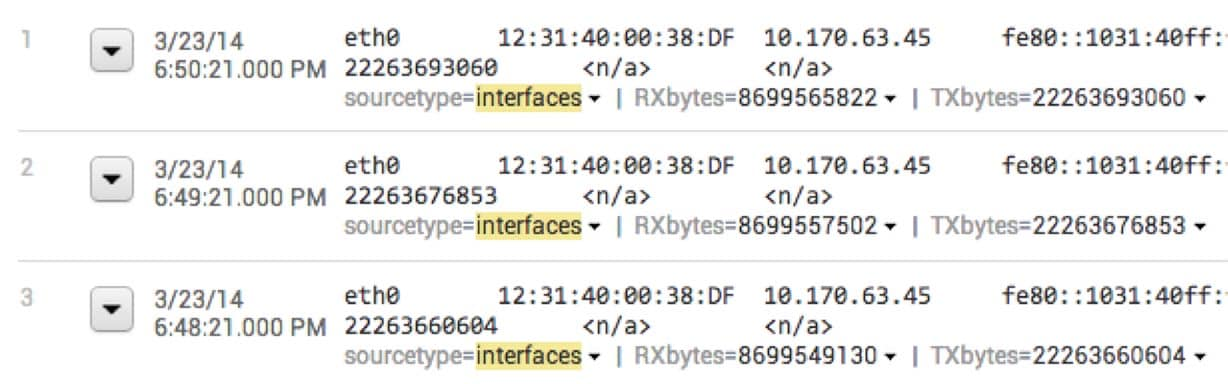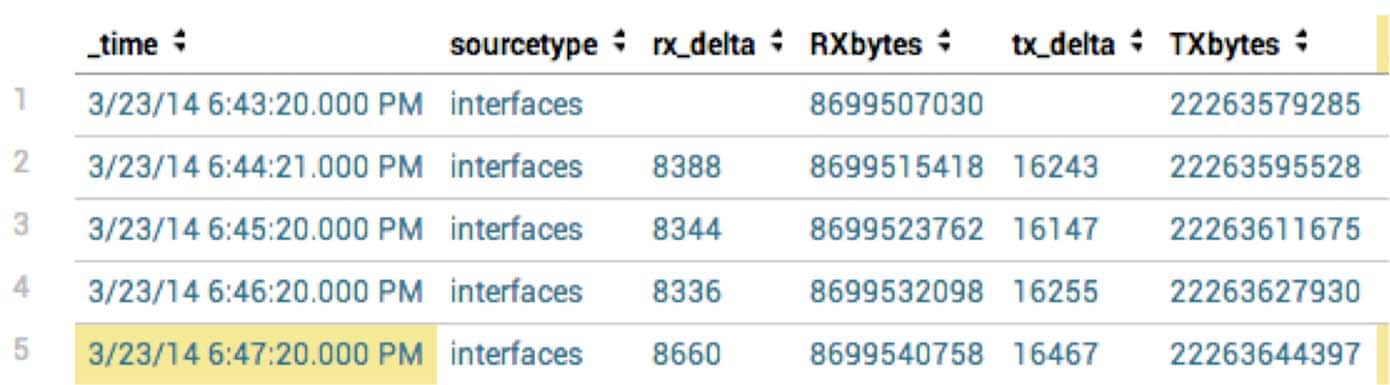TIPS & TRICKS

Search commands > delta

So after my last post discussing accum, I figured I would describe it’s close relative delta. Delta is similar to accum.   It’s purpose is to help you calculate the difference between a field’s value in two different events rather than keeping a running total.

I find delta to be the more useful of the two commands.

Delta:

The syntax for delta is very similar to accum, but it has one additional parameter:

delta (field [AS newfield]) [p=int]

Like accum, the delta command is designed to work on nearby events.  Rather than a running total, delta calculates the difference between field values.  The field parameter tells delta which field to use to calculate the difference and then also allows you to optionally rename the output as a new field.

The one twist is that the delta command also gives you an optional parameter “p”, which lets you use a value other than the previous event for computing the difference.  For example, p=2 would use the value before the previous event.

One common use of delta is calculating throughput when you have a cumulative total value in your events.  For example, in the Splunk app for Unix the interfaces sourcetype has data like below.You can see that RXbytes and TXbytes are the incrementing sum of bytes transferred on any given interface.  If we want to calculate throughput, we just need to know the number of bytes transferred for a given period and divide it by the amount of time it took.  Simple!

To calculate the difference, one method would be to use the delta command.

index=os sourcetype="interfaces" |reverse | delta RXbytes as rx_delta | delta TXbytes as tx_deltaNow you have this the delta calculated, you can easily turn this into a timechart showing bytes transferred per second.  However, forget about dividing it by hand, the timechart command conveniently has per_* aggregators that save you the effort of doing the math!

| timechart per_second(rx_delta) per_second(tx_delta)

In the start of my posts for delta and accum I mentioned that you can often accomplish the same goal in multiple ways with Splunk.  Last time I gave an example for the command streamstats for calculating sums. Streamstats can also be used to calculate the difference between events!

The delta example could be accomplished by using the range aggregator and setting the window.

| streamstats range(RXbytes) as rx_delta range(TXbytes) as tx_delta window=2

Happy Splunking!Posted by